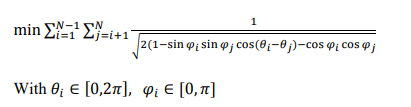# How to set a Non-linear function with double summatory

Hello Again!
I’m trying to solve this optimization problem:But i’m not really sure about how to write down that objective function, I tried doing this:

``````using JuMP
using Ipopt

model = Model(Ipopt.Optimizer)
set_attribute(model, "print_level",4)
set_optimizer_attribute(model, "max_iter",20000)
set_attribute(model, "print_timing_statistics","yes")

n = 32 #number of charges

@variables(model, begin
-1 ≤ x[1:n] ≤ 1
-1 ≤ y[1:n] ≤ 1
-1 ≤ z[1:n] ≤ 1
end)

@NLobjective(model, Min, sum(sum(1/sqrt((x[i] - x[j])^2 + (y[i] - [j])^2 + (z[i] - z[j])^2) for j=i+1:n) for i=1:n-1 ))
optimize!(model)

``````

It’s slightly different since I’m not using spherical coordinates, but what really matters is how to set the objective function with the double summatory.
This will be used for generating 32 equidistant points over an unitary sphere surface.
How do you all recommend me to solve this optimization problem with JuMP ?

1 Like

Looks like you missed a `y`: `y[i] - [j]`. Otherwise, I think your code should work. You could probably drop `@NLobjective` and use `@objective` directly if you’re on JuMP 1.15.

Also, you could probably write with a single sum:

``````d(x, y, z, i, j) = 1 / sqrt(...)
@objective(model, Min, sum(d(x, y, z, i, j) for i = 1:n, j = 1:n if j > i))
``````
2 Likes

You also need to be careful, because when `x[i] == x[j]` (e.g., if all variables are `0`), then the objective is `1 / sqrt(0)` which is undefined. You need to ensure that the objective value is always defined, so maybe use something `1 / sqrt(0.001 + ...)`?

1 Like

It worked, thank you very much

1 Like

I’ll check it, thank you Odow

1 Like